Next: Hyperelastic and hyperfoam materials Up: Materials Previous: Linear elastic materials for   Contents

### Ideal gas for quasi-static calculations

A special case of a linear elastic isotropic material is an ideal gas for small pressure deviations. From the ideal gas equation one finds that the pressure deviation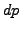is related to a density change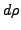by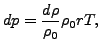(260)

where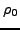is the density at rest,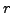is the specific gas constant and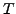is the temperature in Kelvin. From this one can derive the equations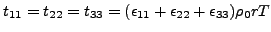(261)

and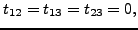(262)

where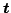denotes the stress and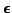the linear strain. This means that an ideal gas can be modeled as an isotropic elastic material with Lamé constants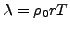and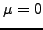. This corresponds to a Young's modulus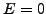and a Poisson coefficient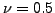. Since the latter values lead to numerical difficulties it is advantageous to define the ideal gas as an orthotropic material with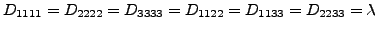and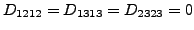.Next: Hyperelastic and hyperfoam materials Up: Materials Previous: Linear elastic materials for   Contents
guido dhondt 2018-12-15